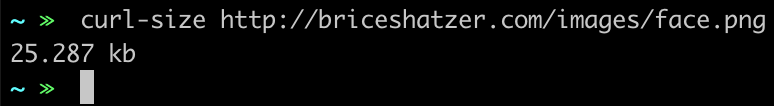April 25, 2019

## Check a File's Size With This Simple Command-line Function

I recently had a project dealing with image compression/hosting and I wanted a way to check the size of a hosted file that was quicker than digging into the network panel or opening Postman each time.

So, I wrote a simple function that I’ve added to my .bashrc file to do exactly that.

Basically it is just a simple wrapper around curl that:

1. Uses `curl -I` to fetch the header of a user provided URL
2. Checks to make sure the header returned useful information
3. Takes that useful header information and formats it in a way that is easy to understand.

### Code

``````function curl-size {
if [[ -z \$1 ]]; then
printf '%s\n' "Please provide a URL"
else
local response=\$(curl -sI "\$1" | tr -d '\r' )
local statusCode=\$(echo \$response | awk '/^HTTP/ {print}')
local byteLength=\$(echo \$response | awk '/[cC]ontent-[lL]ength/ {print \$2}')
if [[ -z \$response ]]; then
printf '%s\n' "Please provide a valid URL"
else
local disposition=UNKNOWN

if [[ \$statusCode = *"200"* ]]; then
disposition=OK
elif [[ \$statusCode = *" 30"* ]]; then
disposition=REDIRECT
local newLocation=\$(echo \$response | awk '/^[lL]ocation:/ {print \$2}')
print \$statusCode
else
disposition=OTHER
fi

function _doMath {
divisor=\$1
print "scale=3;\$byteLength/\$divisor" | bc -l
}

if [[ \$disposition == REDIRECT ]]; then
printf 'redirecting to %s\n' \$newLocation
curl-size \$(echo \$newLocation)
elif [[ \$disposition == OTHER ]]; then
print \$statusCode
else
local value=""
local unit=""
if [[ -z \$byteLength || (\$byteLength == "0") ]]; then
unit="Please provide a valid URL"
elif ((\$byteLength>1000000000));then #1*10^9
value=\$(_doMath 1000000000)
unit="gb"
elif ((\$byteLength>1000000));then #1*10^6
value=\$(_doMath 1000000)
unit="mb"
elif ((\$byteLength>1000));then
value=\$(_doMath 1000)
unit="kb"
else
value="\$byteLength"
unit="bytes"
fi

printf '%s\n' "\$(printf '%s\n' "\$value" | grep -o '.*[1-9]') \$unit"
fi
fi
fi
}
``````

### In Use

Using it for it’s original purpose…It even handles URLs that return non-200 status codes…« All Posts